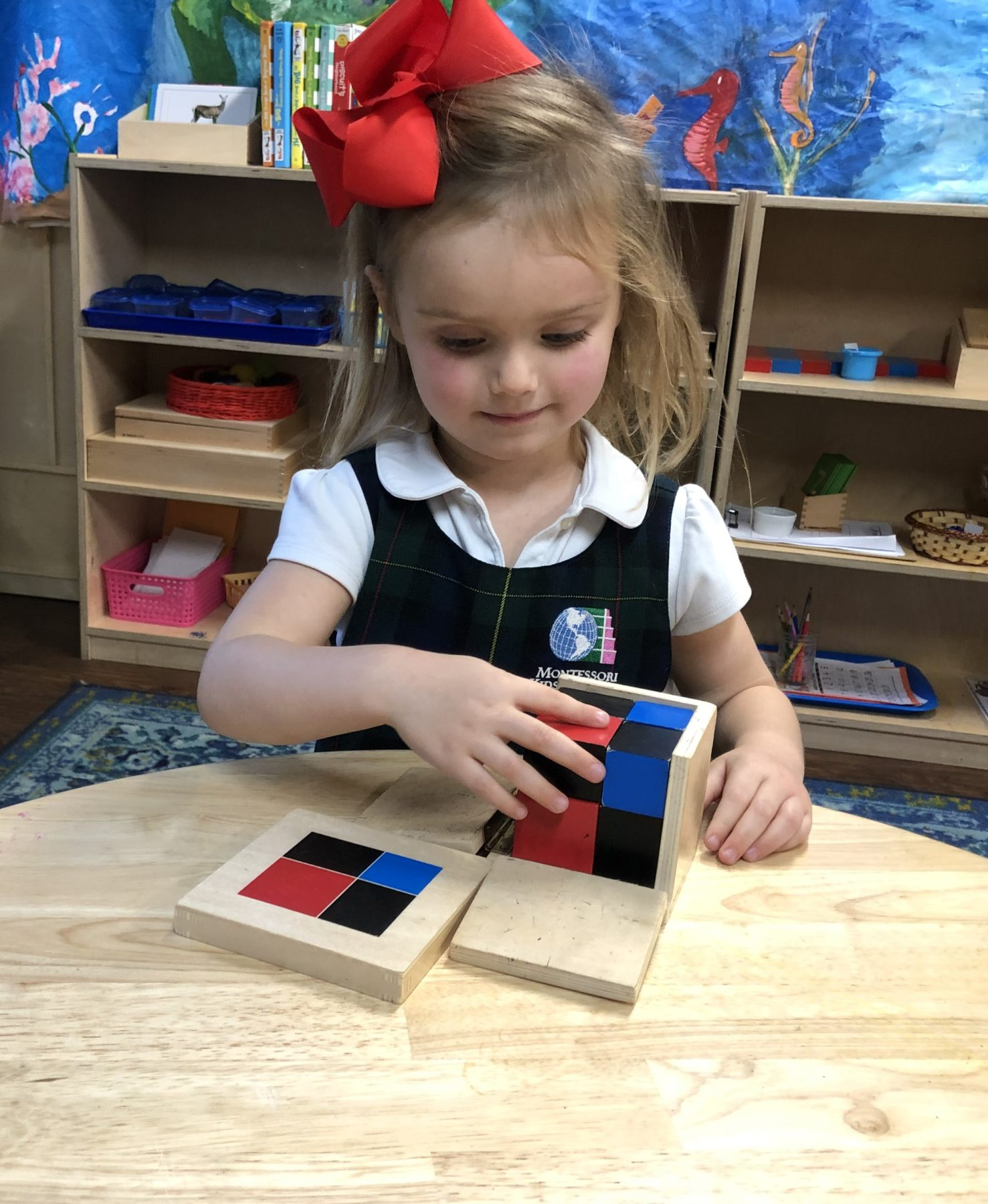Home / Montessori Binomial Cube# Montessori Binomial CubeCan a 3-year-old really learn algebra? Absolutely! In Montessori, we introduce algebra indirectly through our sensorial materials. Many people assume the binomial cube is part of the mathematics curriculum, but it is actually part of the sensorial curriculum with an indirect introduction to the algebraic equation (a+b)3Maria Montessori created sensorial work to help children develop their logic, perception, and awareness skills. The binomial cube does just that by allowing the child a tactile way to sense the differences between the blocks.

What exactly is the Montessori Binomial Cube?

The Montessori binomial cube consists of 8 wooden blocks that fit together in a specific and intentional way into a wooden box with the display of the binomial shown on top. The 8 wooden blocks include one red cube, three red and black matching prisms, one blue cube and three blue and black matching prisms.

What is the purpose of the Montessori Binomial Cube?

All Montessori materials have both direct and indirect aims. The direct aim of the Montessori binomial cube is for the student to properly build the cube within the box while using and developing dexterity and visual acuity. The indirect aim of the binomial cube is to prepare the student to learn advanced algebraic concepts. It is best to allow the child to think of the binomial cube as a 3-D puzzle allowing them to focus on the pattern and spatial relationship instead of worrying about the mathematic formula.

The Montessori binomial cube is one of many sensorial lessons that indirectly prepares the child for mathematics. It is a perfect example of how Maria Montessori introduced concrete learning before abstract learning. Some of the sensorial lessons a child might learn before the binomial cube are geometric cabinet and color tablets There are also many extensions with the binomial cube and once it is mastered children move on to the trinomial cube.